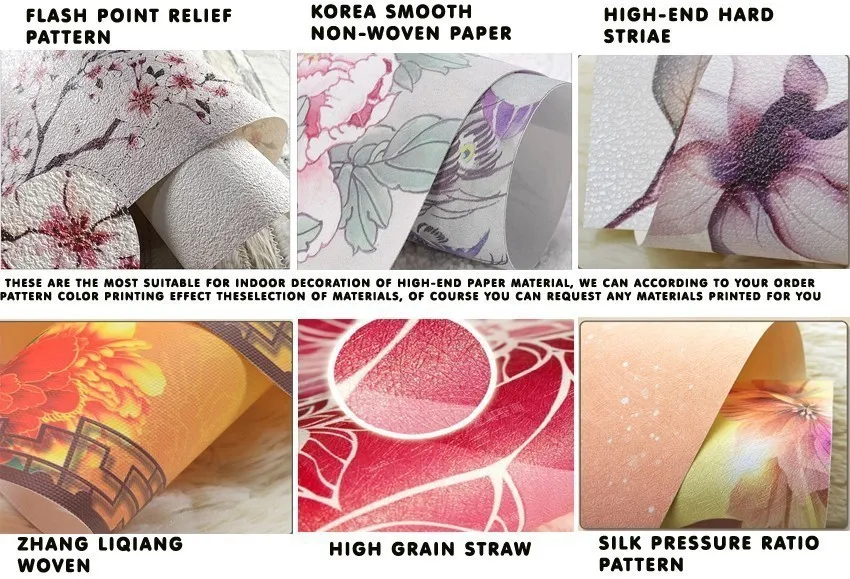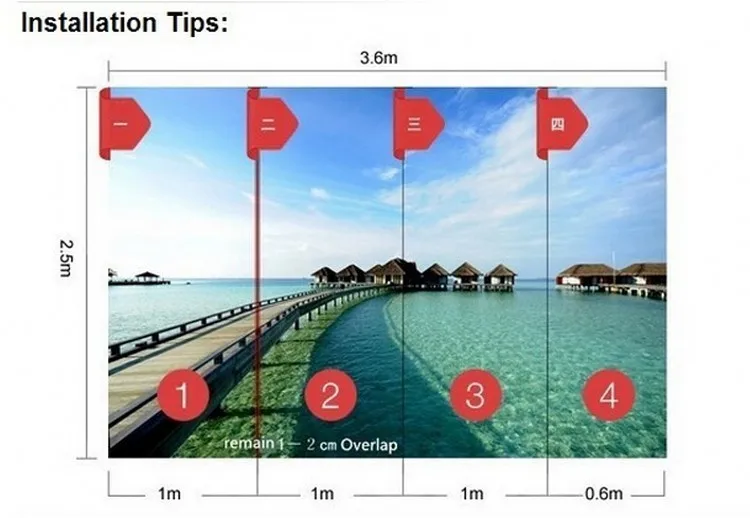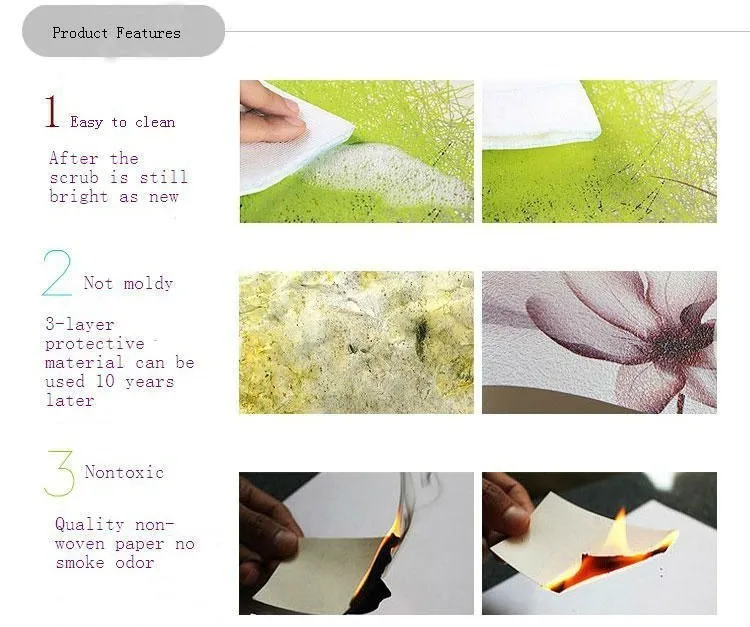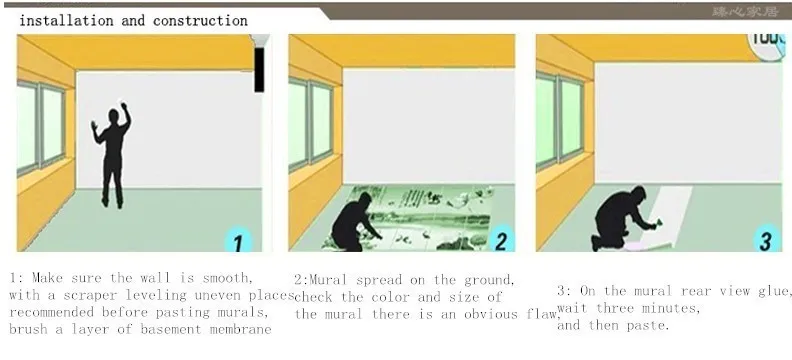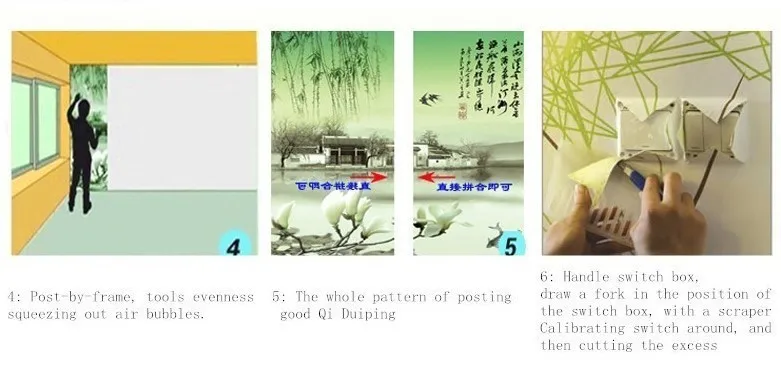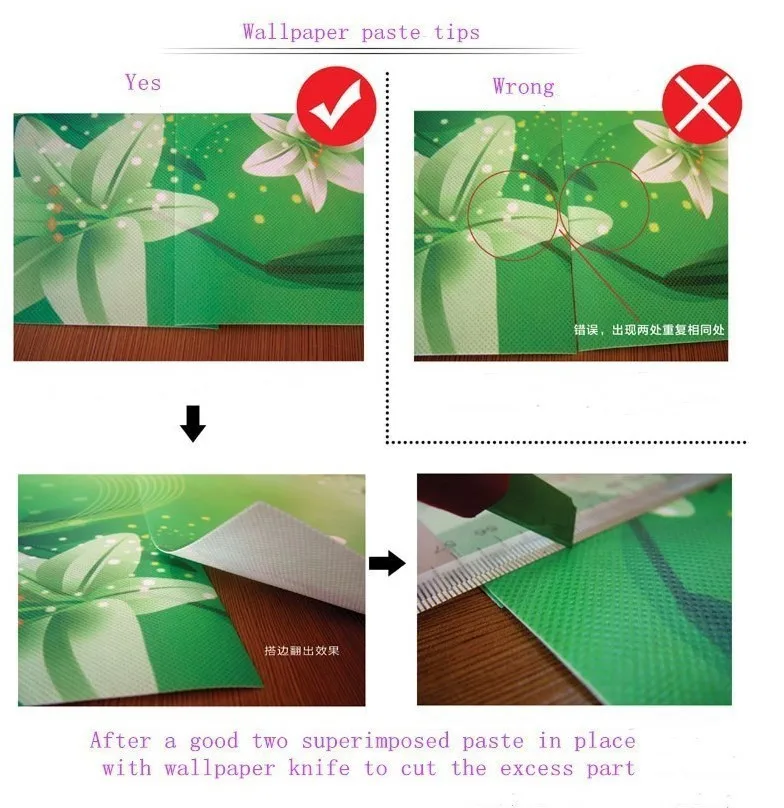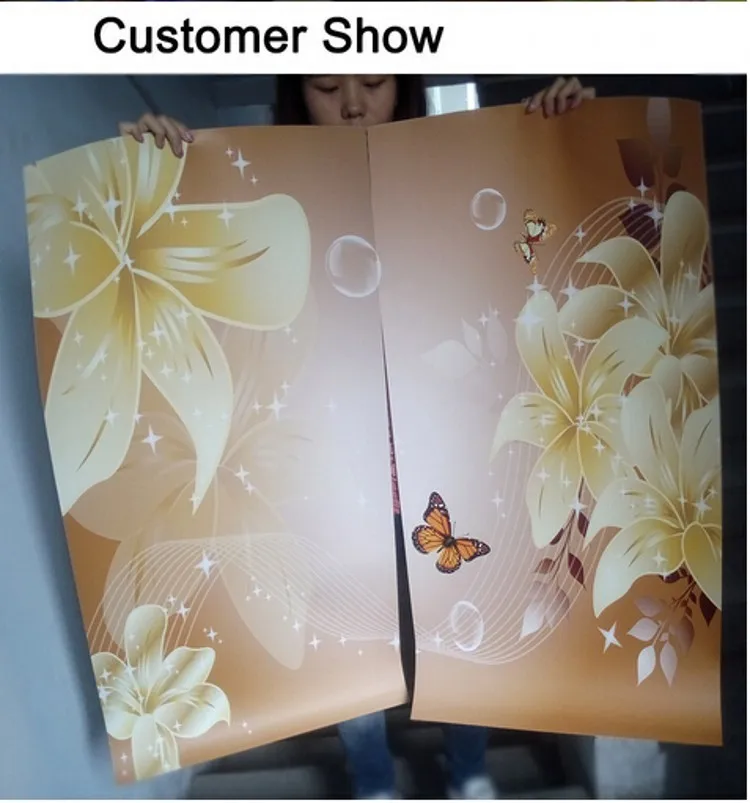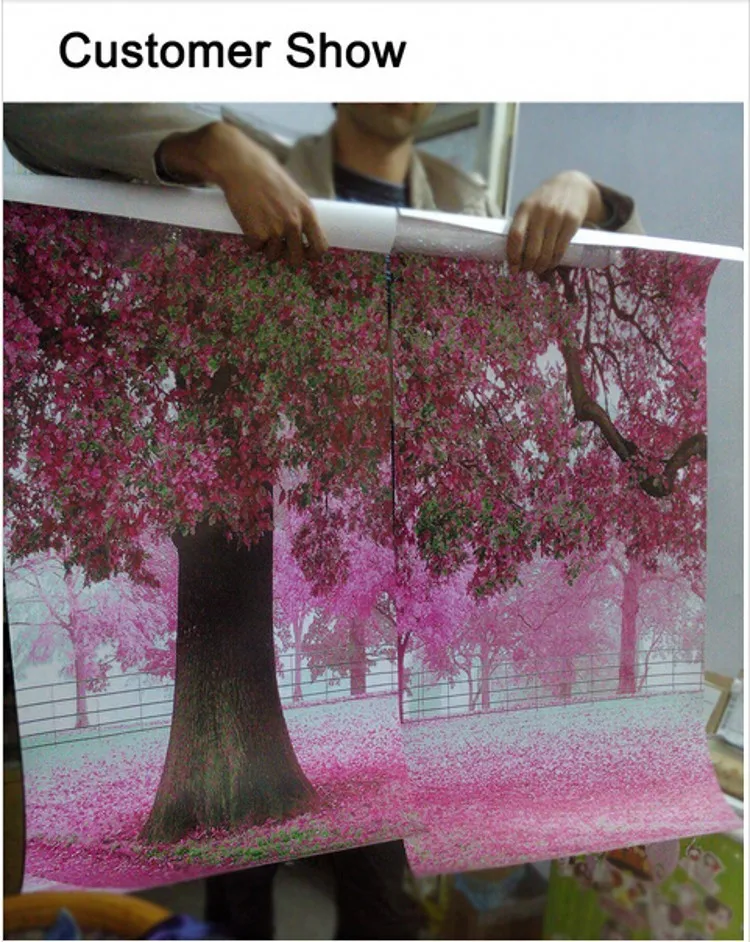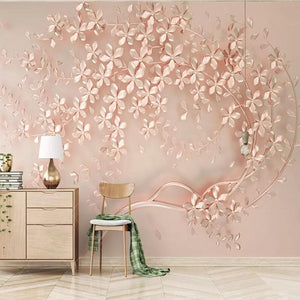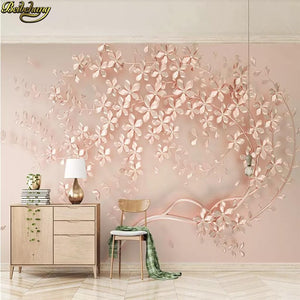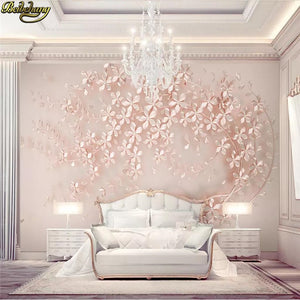# Just Pink Collection - Elegance Rose Gold Flower Wallpaper

Regular price
\$30.19
Sale price
\$30.19
Regular price
\$46.45
Sold out
Unit price
per
Shipping calculated at checkout.

mural wallpaper price: \$ / square meter.    Not rolls.

square meter = 1pcs.   2 square meter=2pcs.   3 square meter =3pcs ...

Width x height = square meters = Order Quantity

Note: Please be careful to order 1m2. Because a very small size

We have 12 fixed size order.

Quantity: 1  = 1 s    quare meter         = 140cm (W) x 70cm (H) (4'3 "x 2'3") ft

Quantity: 2  = 2 s    quare meter         = 200cm (W) x 100cm (H) (6'5 "x 3'2") ft

Quantity: 3  = 3     s    quare meter         = 208cm (W) x 146cm (H) (6'8 "x 4'8") ft

Quantity: 4  = 4     s    quare meter         = 200cm (W) x 200cm (H) (6'5 '' x 6'5 '') ft

Quantity: 5  = 5      s    quare meter         = 250cm (W) x 200cm (H) (7'6 '' x6'5 '') ft

Quantity: 6 = 6      s    quare meter         = 300cm (W) x 200cm (H) (9'8 '' x 6'5 '') ft

Quantity: 7 =7     s    quare meter         = 300cm (W) x 250cm (H) (9'8 "x 8'2") ft

Quantity: 8 =8      s    quare meter         = 400cm (W) x 200cm (H) (13'6 "x 6'5") ft

Quantity: 9  =9       s    quare meter         = 350cm (W) x 260cm (H) (11'4 "x 8'7") ft

Quantity: 10 = 10      s    quare meter         = 360cm (W) x 280cm (H) (11'8 "x 9'2") ft

Quantity: 12 =12      s    quare meter         = 400cm (W) x 300cm (H) (13'6 "x 9'8") ft

Quantity: 16 =16      s    quare meter         = 500cm (W) x 320cm (H) (16'4 "x 10'5") ft

Other size . (width and height) . please tell us.

We will give you calculate the correct order quantity and price

FAQ: buyers ordered to leave. No size information. what to do?

We will wait for buyers 24 hours.

We will send          corresponding          default 12 fixed size.

FAQ:How to order other sizes?

Order other Size steps:

1: Select the correct quantity = square meter (width x height)

3: Fill in details .In the order number inside, write your size        information

4: Submit your order .Payments .on OK

Width x height = square meters (square feet)

1 square meter=10.76 square feet

tip:If the feet (inches) Give me information I will give you calculate the correct order quantity

For example         : You need to wall :Width = 2.8m  Height = 1.7m..

2.8m x 1.7m = 4.76m2 rounding .You need to order Quantity: 5

FAQ: What this wallpaper?

This is the photo wallpaper. Mural wallpaper. wall mural.

Not wallpaper rolls. Not a poster. Not wall stickers.

FAQ: How do I know the size of the photo wallpaper?

This is a custom wallpaper. Not 10m L and 0.53m W wallpaper rolls

FAQ: How to paste this wallpaper?

Paste it. With the same wallpaper rolls. It needs adhesive paste

FAQ: How to Order size of the main picture?

You need to wall size. Width and height. Please tell us information

FAQ: Any size is the overall pattern?

Yes.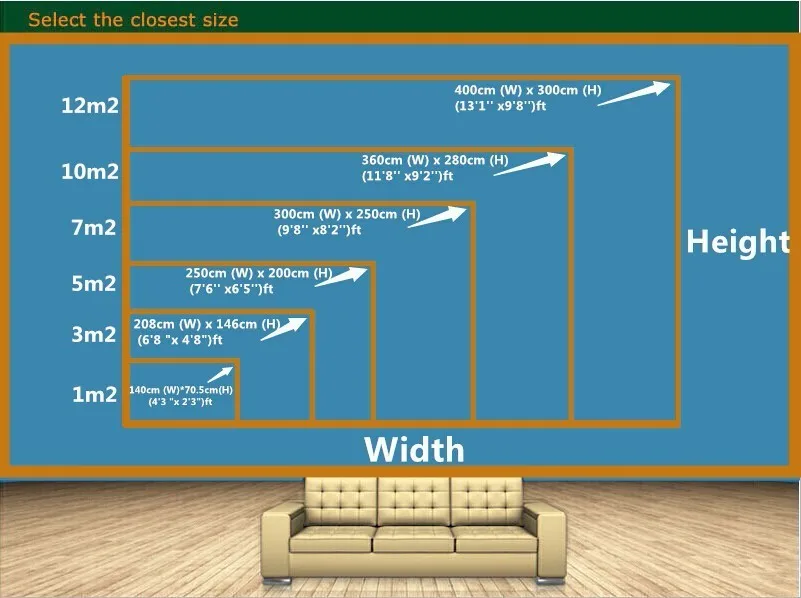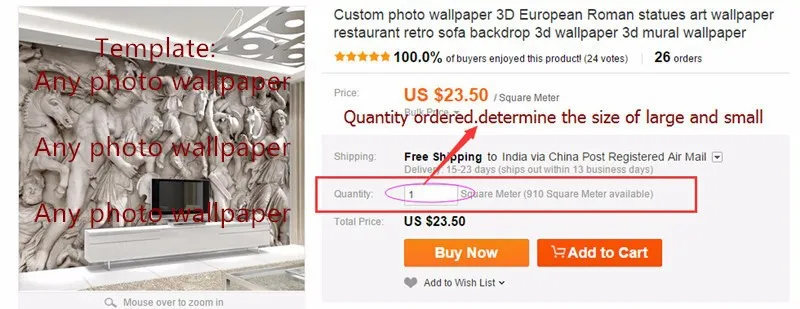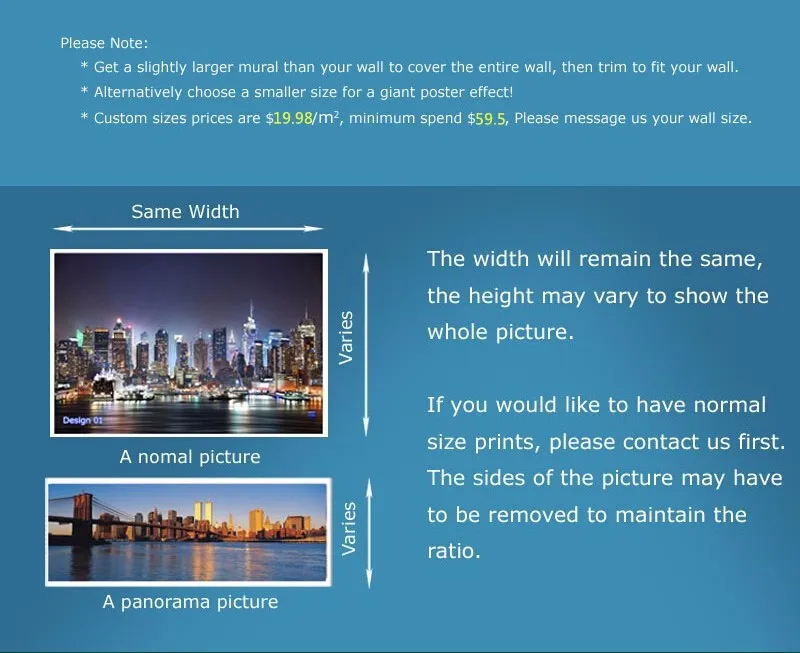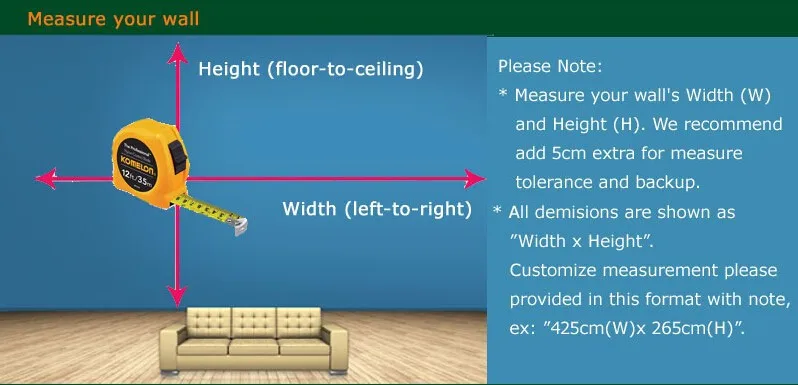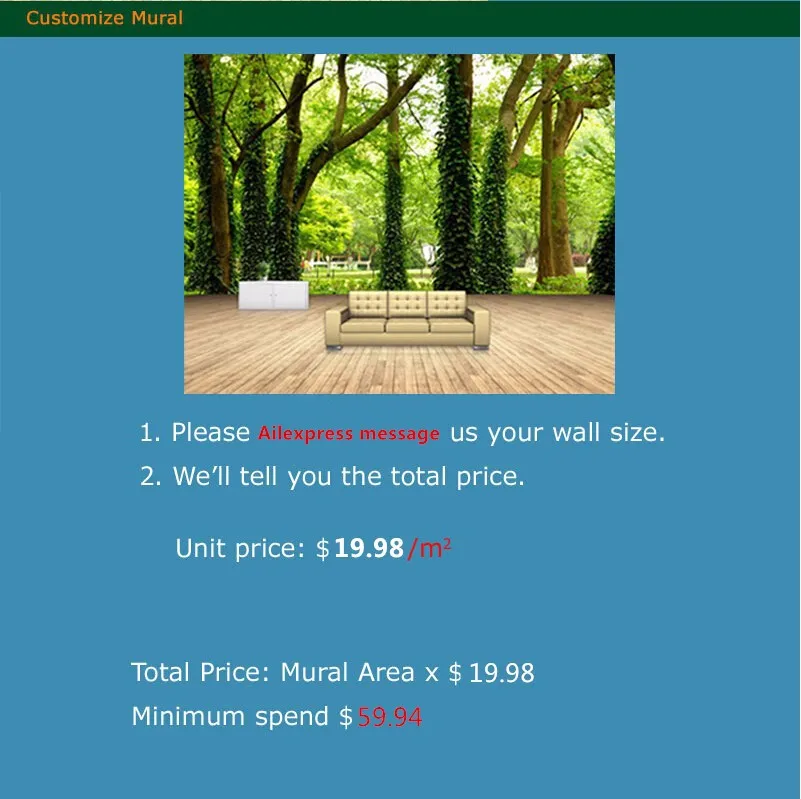Kind shown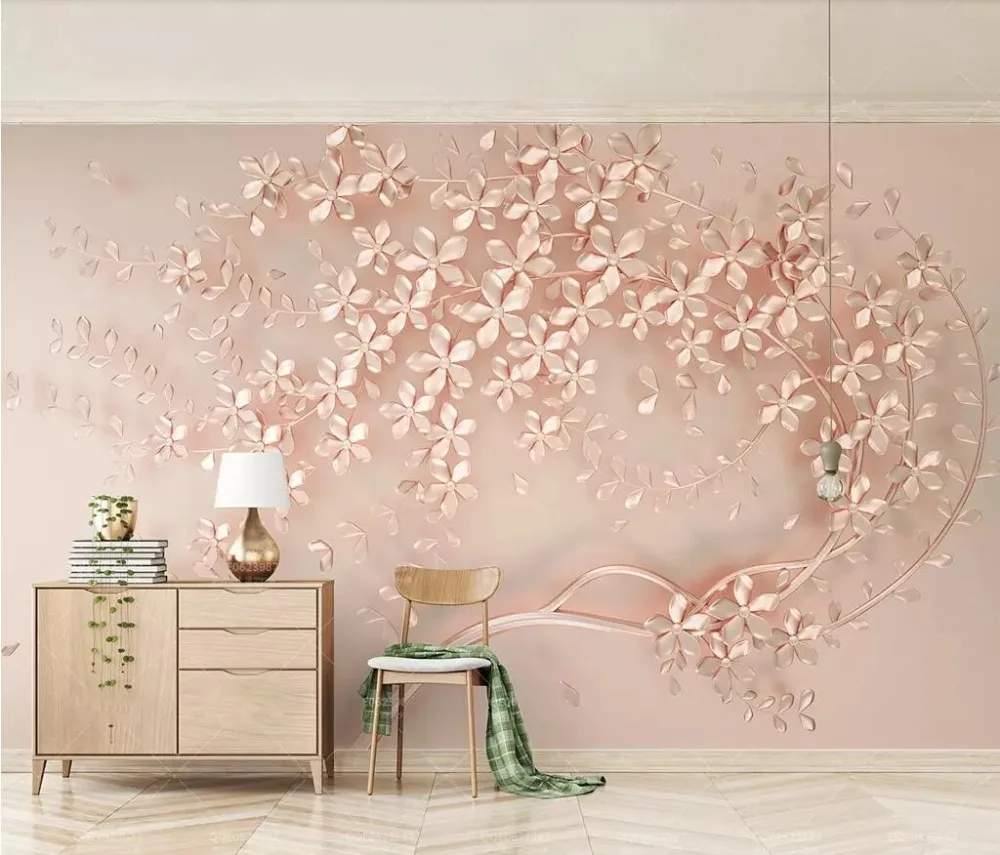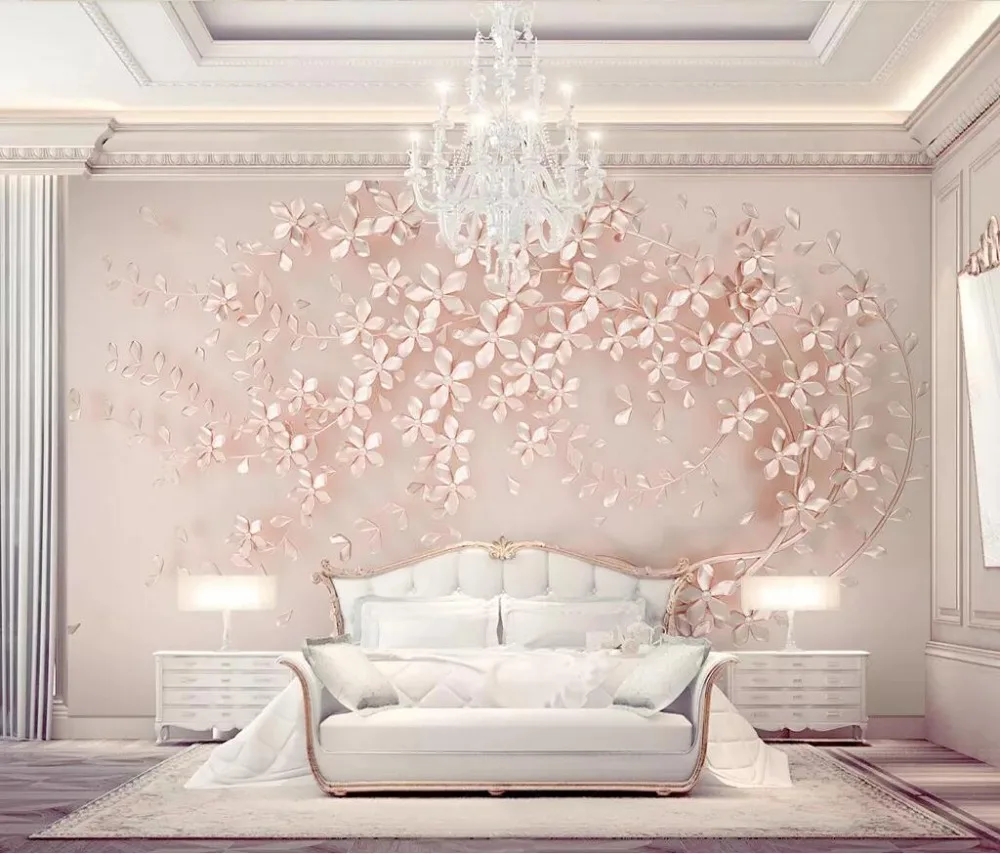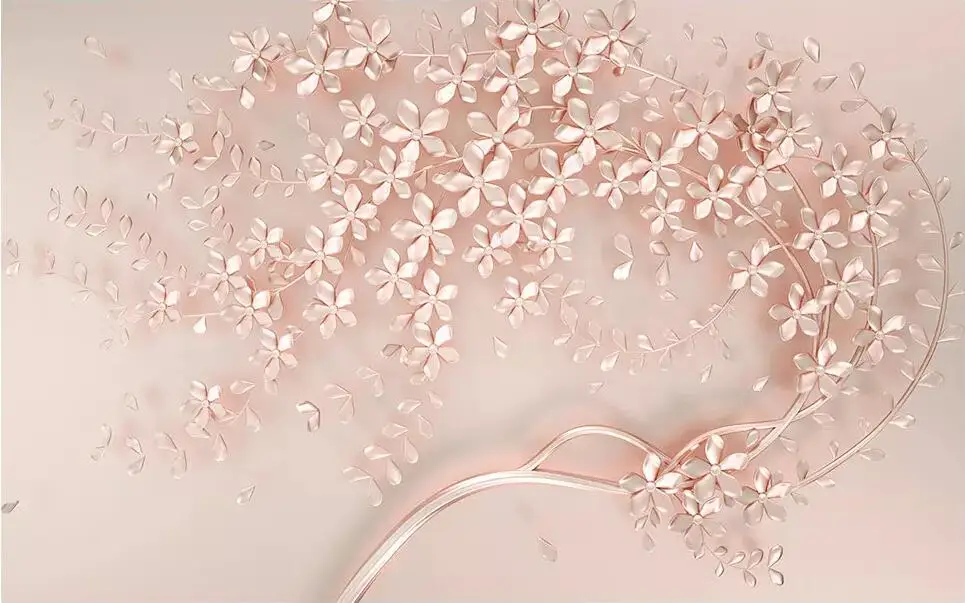Product Description